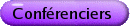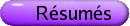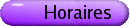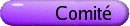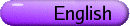Géométrie discrète et convexe
Org: Karoly Bezdek (Calgary) et Jozsef Solymosi (UBC)
[PDF]

KAROLY BEZDEK, University of Calgary, 2500 University Drive NW, Calgary, Alberta T2N 1N4
Antipodality revisited
[PDF]

Antipodality is an important concept in discrete geometry with a variety of meanings. The talk surveys some of the most recent developments.

KAROLY BOROCZKY, Eotvos University, Budapest, Pazmany Peter s. 1/C, 1117 Hungary
Transversals of hyperbolic disks
[PDF]

According to the classical result of Hadwiger, if any three discs have a transversal in an infinite packing of congruent disks in the Euclidean plane then the whole family has a transversal. I prove that if any three sets have a transversal in an infinite packing of connected sets with uniformly bounded diameters in the hyperbolic plane then the whole family has a transversal.

Next, Danzer verified that if any five discs have a transversal in a finite packing of congruent disks in the Euclidean plane then the whole family has a transversal. I prove the analogous statement in the hyperbolic plane for disks and for horodisks. In addition I exhibit packings of arbitrary finite number of congruent disks in the hyperbolic plane such that any four has a transversal but the whole family has not. Moreover we exhibit packings of arbitrary finite number of congruent disks in the hyperbolic plane such that any three has a transversal but removing any disk from the family, even the rest has no transversal.

BOB ERDAHL, Queen's University
Delaunay Polytopes and the Pauli Principle
[PDF]

Consider the integer lattice Zd, and a lattice polytope P in Zd. Then, P is Delaunay if it can be circumscribed by an empty ellipsoid E, where there are no lattice points interior to E, and the only Zd-elements on the boundary are the vertices of P. The empty ellipsoid E determines an inhomogeneous quadratic function up to a scale factor. In general there are many empty ellipsoids that circumscribe P, each determining a single ray of quadratic functions. The collection of all such rays is a relatively open cone of quadratic functions, the inhomogeneous domain D(P), of the Delaunay polytope P.

If the vertices of P are (0,1)-vectors, then these can be interpreted as giving partial information on a quantum system of electrons that are distributed amongst d one-particle states. Each (0,1)-vector is a vector of occupation numbers, component k telling whether state k is occupied or unoccupied; the occupation numbers are limited to 0 or 1 by the Pauli principle. By taking averages we have a more general situation; the components belong to the unit interval and give the probability that state k is occupied. With this quantum interpretation each quadratic function in the domain D(P) corresponds to a Hamiltonian operator on the state space of the quantum system, and the vertices of the Delaunay polytope label a basis for the degenerate ground state of this Hamiltonian.

Besides the probability distribution for the electrons, it is also useful to determine the joint probability distributions for pairs of electrons, and possibly even higher order distributions of electrons. I will describe how pair probability distributions can be described within the present framework, and how the convex set of all pair probability distributions can be described using the family of operators corresponding to the elements of D(P). I will relate this discussion to some questions in quantum information theory.

FERENC FODOR, University of Szeged, Bolyai Institute, 1 Aradi vértanúk tere, 6720 Szeged, Hungary
Lower bounds on the surface area of Voronoi polyhedra
[PDF]

One of the most beautiful problems related to 3-dimensional unit ball packings is the Dodecahedral Conjecture formulated by L. Fejes Tóth in 1943. It states that the minimal volume of a Voronoi cell in a 3-dimensional unit ball packing is at least as large as the volume of a regular dodecahedron of inradius 1. This problem has been recently settled in the affirmative by Hales and McLaughlin. K. Bezdek phrased the following generalized version of the Dodecahedral Conjecture in 2000. Strong Dodecahedral Conjecture: The minimum surface area of a Voronoi cell in a unit ball packing in E3 is at least as large as the surface area of the regular dodecahedron circumscribed about the unit ball.

In this talk, I will present a construction which yields a lower bound on the surface area of the Voronoi polyhedron. This result improves on existing lower bounds and provides further support for the Strong Dodecahedral Conjecture.

This work is joint with Gergely Ambrus.

MARINA GAVRILOVA, University of Calgary, 2500 University Drive NW, Calgary, AB T2N 1N4
Exact Point Location in Generalized Voronoi Diagram
[PDF]

The talk is devoted to the problem of exact point-location in a generalized d-dimensional Voronoi Diagram in the Euclidean space. The exact point location problem typically requires the solution for expressions of degree four. An approximation of the solution using using expression of a smaller degree is possible through polyhedral metrics. In general dimensions two Minkowski metrics can be used (Manhattan and supremum). The computation uses degree one. We also show that a polygonal metric can be applied in two dimensions. The computation involves only O(lg k) calls of the algorithm ESSA for detecting the sign of a sum using floating-point arithmetic.

MOHAMMAD GHOMI, Georgia Institute of Technology, Atlanta, Georgia 30308
Isoperimetric inequality outside convex bodies
[PDF]

We prove that the area of a hypersurface which traps a given volume outside of a convex body in Euclidean n-space must be greater than or equal to the area of a hemisphere trapping the given volume on one side of a hyperplane. This result generalizes the classical isoperimetric inequality. The proof rests on a sharp estimate for total positive curvature of a hypersurface whose boundary lies on a convex body and meets that body orthogonally.

This is joint work with J. Choe and M. Ritore.

LUIS GODDYN, Simon Fraser University
Log-discrepancy and chromatic number of hyperplane arrangements
[PDF]

To sign an arrangement of affine hyperplanes in Rd is to to designate, for each hyperplane, one of its two sides as being "positive". A vertex is any intersection of d affinely independent hyperplanes. We seek a signing for which each vertex lies in the positive side of about 50% of the hyperplanes not containing the vertex. This differs from "discrepancy theory", where the goal is to minimize the difference rather than the ratio between the number of positive and negative sides containing each vertex. Hence the phrase "log-discrepancy" in the title.

An old formula of Minty expresses the (circular) chromatic number of a graph in terms of finding a signing of low log-discrepancy in an associated complex. This motivates the invariant we study here. It also allows us to define a "chromatic number" for any hyperplane arrangement and, more generally, for any oriented matroid.

Using a probabilistic argument, we prove that the chromatic number of any loopless oriented matroid is bounded above by a function of its corank. This generalizes the observation that the chromatic number of a loopless connected graph (V,E) is bounded above (approximately) by the square root of its Beti number |E| - |V| + 1.

This is joint work with P. Hlineny and W. Hochstaettler.

BRANKO GRÜNBAUM, University of Washington, Seattle, WA 98195-4350, USA
Enumeration of square-faced isogonal infinite polyhedra
[PDF]

The three well-known Coxeter-Petrie polyhedra are examples of regular infinite polyhedra with convex faces. This means that the symmetry group of each acts transitively on its flags, which implies that all faces are congruent regular polygons and that all vertices are equivalent under symmetries of the polyhedron. In the finite case the implication can be reversed resulting in the five Platonic solids. Not so in the infinite case. Adding to previously known results for the infinite case, obtained by various authors, we completely enumerate the infinite polyhedra with square faces, with adjacent faces either coplanar or perpendicular, and with all vertices equivalent under symmetries. There are precisely 15 such polyhedra in which each vertex is incident with five squares, and 6 in which each is incident with six. These enumerations are accomplished via a combination of combinatorial and geometric calculations, both manual and computerized, along with computer graphics visualizations of the polyhedra. The method can be used in more general situations as well.

The work is a collaboration of Steven Gillispie and the presenter, both at the University of Washington.

Near-transversal lines in families of congruent circles
[PDF]

Let F be a family of congruent circles. We say that F has property T(3) if every triple of its members has a line transversal. In 1980, Katchalski and Lewis proved the existence of a constant m such that in every T(3)-family of disjoint congruent discs there is a line intersecting all but at most m of the discs. A family of congruent circles of unit diameter is said to be t-disjoint if the distance of every pair of the circles is larger than t. We have two generalizations of the above mentioned Katchalski-Lewis theorem:

(1) The theorem holds with the conjectured m = 2.
(2) The theorem holds for t-disjoint families as well: To every t > 0 there exists a constant m(t) such that in every T(3)-family of t-disjoint congruent discs there is a line intersecting all but at most m(t) of the discs.

GYORGY KISS, Eötvös Loránd University, 1117 Budapest, Pázmány s. 1/c, Hungary and University of Szeged, 6720 Szeged, Aradi vértanúk t. 1, Hungary
On the illumination parameters of convex bodies
[PDF]

Let K be a convex body of Ed. We say that a point, i.e., a light-source l, illuminates the boundary point p of K if the half-line starting at l and passing through p intersects the interior of K. Furthermore we say that the light-sources {l1,l2,...} Ì Ed \K illuminate K if each of its boundary points is illuminated by at least one of the light-sources {l1,l2,...}. The well-known illumination conjecture of Hadwiger (1960) says that any d-dimensional convex body can be illuminated by 2d light-sources. In this talk we consider some quantitative versions of Hadwiger's problem. If B is a centrally symmetric body about the origin o of Ed, then let the illumination parameter of B be defined as

 b(B) = inf ìí î å i d(o,li) : {li} illuminates B üý þ .
This ensures that far-away light-sources are penalised. K. Bezdek proved (1992) that b(B) < 6 holds in E2. He also proved (2005) that b(B) = 6Ö3 if B is the unit ball E3.

We prove some theorems about the illumination parameters in E3, and give some possible generalizations in Ed, when we consider the illumination by r-dimensional linear light-sources instead of points.

KEE YUEN LAM, University of British Columbia
The combinatorics of sums of squares as studied via topology
[PDF]

Historically, the well-known identity

 (x12 + x22) (y12 + y22) = (x1 y1 - x2 y2)2 + (x1 y2 +x2 y1)2
had led to a "sums of square" problem, namely to determine all identities of the type
 (x12 + ... + xr2) (y12 + ... + ys2) = f12 + ... +fn2
where f1,...,fn belong to a polynomial ring L[x1,...,xr; y1,...,ys]. In this generality the problem remains wide open. For the case when the commutative ring L is Z, it reduces to a question of discrete mathematics, involving "signed intercalate matrices". In this talk I shall explain what intercalate matrices are, and show how ideas from algebraic topology can be used in the study of the combinatorics of such matrices.

HIROSHI MAEHARA, University of the Ryukyus, Nishihara, Okinawa, 903-0213 Japan
Reversing a polyhedral surface
[PDF]

We introduce a new variety of flexatube, a rhomboflexatube. It is obtained from a cardboard rhombohedron by removing a pair of opposite faces (rhombi), and then subdividing the remaining four faces by pairs of diagonals. It is reversible, that is, it can be turned inside out by a series of folds, using edges and diagonals of the rhombi. To turn a rhomboflexatube inside out is quite a challenging puzzle. We also consider the reversibility of general polyhedral surfaces. We show that if an orientable polyhedral surface with boundary is reversible, then its genus is 0 and for every interior vertex, the sum of face angles at the vertex is at least 2p. After defining tube-attachment operation, we show that every polyhedral surface obtained from a rectangular tube by applying tube-attachment operations one after another can be subdivided so that it becomes reversible.

EGON SCHULTE, Northeastern University, Department of Mathematics, Boston, MA 02115
Local Theorems in Combinatorial Tiling
[PDF]

A locally finite face-to-face tiling of euclidean d-space by convex d-polytopes is said to be combinatorially crystallographic if its combinatorial automorphism group has only finitely many orbits on the tiles. We describe a new Local Theorem, which characterizes combinatorially crystallographic tilings in terms of large enough neighborhood complexes (centered coronas) of tiles. This generalizes the Local Theorem for Monotypic Tilings, which gives necessary and sufficient conditions for combinatorial tile-transitivity.

Both results are joint work with Nikolai Dolbilin.

JOZSEF SOLYMOSI, University of British Columbia
The geometry of Schur's theorem
[PDF]

One of the first results in additive combinatorics belongs to I. Schur and dates back to 1916. His motivation was to study "the local version" of the famous equation of Fermat: xn+yn = zn. If there are integers x, y, z satisfying the above equation, then for every prime p, they also solve the congruence equation: xn + yn º zn (mod p). He showed that the congruence equation has a non-trivial solution for all large primes p. This result follows from the next result, known as Schur's Theorem: Let r > 1. Then there is a natural number S(r), such as if N > S(r) and if the numbers {1,2,...,N} are coloured with r colours, then there are three of them x, y, z of the same colour satisfying the equation: x + y = z. We will give a geometric-combinatorial proof for Schur's Theorem and extend it, to prove a density version, similar to Roth's theorem for 3-term arithmetic progressions.

CSABA D. TOTH, MIT, Cambridge, MA 02144
Spanning trees for disjoint barriers in three-space
[PDF]

Given n point sites and a set of disjoint barriers in three-space, we wish to connect the sites with a straight line spanning tree such that no barrier intersects "too many" edges of the tree. We show that if the barriers are convex 2-dimensional objects of bounded description complexity, then there is a spanning tree such that every barrier cuts at most [(O)\tilde](Ön) of its edges. If the barriers are axis-aligned rectangles, then there is a spanning tree such that every rectangle cuts at most O(n1/3) of its edges. Both bounds are asymptotically optimal.

Joint work with Eynat Rafalin and Diane Souvaine.

ASIA IVIC WEISS, York University, 4700 Keele St., Toronto, Ontario
Petrie-Coxeter maps revisited
[PDF]

A technique is presented for constructing new chiral or regular polyhedra (or maps) from self-dual abstract polytopes of rank 4. From improperly self-dual chiral polytopes we derive "Petrie-Coxeter-type" polyhedra (abstract chiral analogues of the classical Petrie-Coxeter polyhedra) and investigate their groups of automorphisms.